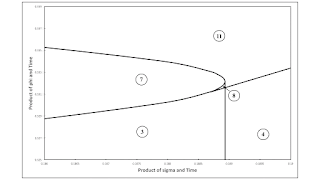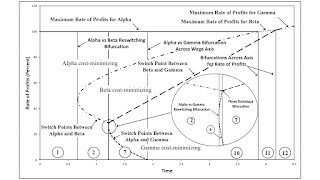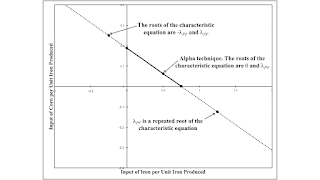## Wednesday, November 29, 2017

### Bifurcation Analysis of a Two-Commodity, Three-Technique Technology

This post expands on this previous post. The technology is the same, but the rates of decrease of the coefficients of production in the Beta and Gamma corn-producing processes are not fixed. Instead, I consider the full range of parameter values. (I find the graphs produced by bifurcation analysis interesting for this case, but I think a two-commodity example can be found with more pleasing diagrams.)

Anyways, Figure 1 shows a bifurcation diagram for the parameter space in this example. The region numbered 8 is not visible on the graph. Accordingly, Figure 2 below shows a much expanded picture of the parameter space around that region. The specific parameter values in the previous post lead to a temporal path along the dashed ray extending from the origin in Figure 1. (The numbering of regions in this post and the previous post do not correspond.) Although it is not obvious, the locus of points bifucating regions 9 and 10 eventually, somewhere to the right of the region shown in Figure 1 eventually decreases in slope and intercepts the dashed ray.Figure 2: Blowup of a Part of the Bifurcation Diagram

As usual, each numbered region corresponds to a definite sequence of cost-minimizing techniques contributing wage curves along the wage frontier. Table 1 lists this sequence for each region. Some notes on switch points are provided. A switch point is called "normal" merely if it conforms to outdated neoclassical intuition. In other words, such a switch point exhibits negative real Wicksell effects. In the example, regions also exist where switch points exhibit positive real Wicksell effects.

 Region Cost-MinimizingTechniques Notes 1 Alpha One technique cost-minimizing. 2 Alpha, Beta "Normal" switch point. 3 Beta, Alpha, Beta Reswitching. Switch pt. athighest r is "perverse". 4 Beta One technique cost-minimizing. 5 Alpha, Gamma "Normal" switch point. 6 Alpha, Gamma, Beta "Normal" switch points. 7 Beta, Alpha,Gamma, Beta Recurrence of techniques.Switch pt. at highest r is"perverse". 8 Beta, Alpha, Beta,Gamma, Beta Two reswitchings, two"perverse" switch pts. 9 Gamma One technique cost-minimizing. 10 Gamma, Beta "Normal" switch point. 11 Beta, Gamma, Beta Reswitching. Switch pt. athighest r is "perverse".

One can compare and contrast the above bifurcation diagram with the one in this post. The latter bifurcation diagram is for a specific instance of the Samuelson-Garegnani model, in which the basic commodity varies among techniques. (I have a more recent write-up of that bifurcation analysis linked to here.)

## Saturday, November 25, 2017

### Reswitching Without a Reswitching Bifurcation

This post presents another example of bifurcation analysis applied to structural economic dynamics with a choice of technique. This example illustrates:

• Two reswitching examples appear and disappear without a reswitching bifurcation ever occurring, at least on the wage frontier.
• Two bifurcations over the wage axis arise. At the time each bifurcation of this type occurs, another switch point for the same techniques exhibits a real Wicksell effect of zero. Thus, for each, a switch point transitions from being a "normal" switch point to a "perverse" one exhibiting capital-reversing.
• Each of the four types of bifurcations of co-dimension one that I have identified have no preferred temporal order. For example, a bifurcation over the wage axis can add a switch point to the wage frontier. And another such bifurcation can remove a switch point, as time advances.
• The maximum rate of profits approaches an asymptote from below as time increase without bound.

Table 1 specifies the technology for this example, in terms of two parameters, σ and φ. Managers of firms know of one process for producing iron and of three processes for producing corn. Each process is defined in terms of coefficients of production, which specify the quantities of labor, iron, and corn needed to produce a unit output for that process. All processes exhibit constant returns to scale; require a year to complete; and totally use up, in producing their output, the capital goods required as input. I consider the special case in which the rate of decrease of the coefficients of production in the Beta corn-producing process, σ, is 5 percent, and the rate of decrease of coefficients in the Gamma corn-producing process, φ, is 10 percent.

 Input IronIndustry Corn Industry Alpha Beta Gamma Labor 1 0.89965 0.71733 e-σ t 1.28237 e-φ t Iron 0.45 0.025 0.00176 e-σ t 0.03375 e-φ t Corn 2 0.1 0.53858 e-σ t 0.13499 e-φ t

Three techniques are available for producing a net output of, say, corn, while reproducing the capital goods used as input. The Alpha process consists of the iron-producing process and the corn-producing process labeled Alpha. And so on for the Beta and Gamma techniques.

The choice of technique is analyzed in the usual way. I assume that labor is advanced, and wages are paid out of the surplus product at the end of the year. Corn is taken as numeraire. A wage curve can be drawn for each technique, given the coefficients of production prevailing at a given moment in time. Figure 1 illustrates a case of the recurrence of techniques in the example. The cost-minimizing technique is found by constructing the outer frontier of the wage curves. In Figure 2, the cost-minimizing techniques are Beta, Alpha, Gamma, and Beta, in that order. The switch point at approximately 57 percent exhibits capital-reversing. Around the switch point, a higher wage is associated with the adoption of a more labor-intensive technique. If prices of production prevail, firms will find it cost-minimizing to hire more workers at a higher wage, given net output.

Figure 3 illustrates the analysis of the choice of technique for all time. Switch points along the frontier and the maximum rate of profits are plotted versus time. Figure 1, at the top of this post, is a blowup of Figure 3 from time zero to a time of five years. These pictures show which technique is cost-minimizing at each rate of profits, at each moment in time. Bifurcations are also shown. Table 2 lists the cost-minimizing techniques in each region between the bifurcations.Figure 3: An Extended Bifurcation Diagram

 Region Cost-MinimizingTechniques Notes 1 Alpha One technique cost-minimizing. 2 Alpha, Beta "Normal" switch point. 3 Beta, Alpha, Beta Reswitching. Switch pt. athighest r is "perverse". 4 Beta, Alpha, Gamma, Beta Recurrence of techniques. Switchpt. at highest r is "perverse". 5 Beta, Gamma, Beta Reswitching. Switch pt. athighest r is "perverse". 6 Gamma, Beta "Normal" switch point. 7 Gamma One technique cost-minimizing.Maximum r approaches anasymptote.

I suppose I can extend this example to partition the complete parameter space, as in this example, with an updated write-up here. That analysis will demonstrate, by example, that this sort of bifurcation analysis applies to cases in which multiple commodities are basic in multiple techniques. It is not confined to the special case of the Samuelson-Garegnani model. I am also thinking that I could perform a bifurcation analysis where parameters that vary include the ratio of the rates of profits in various industries, as in these examples of a model of oligopoly. Maybe such an analysis will yield an empirically relevant tale of the evolution of economic duality (also known as segmented markets).

## Wednesday, November 22, 2017

### Bifurcation Analysis Applied to Structural Economic Dynamics with a Choice of TechniqueVariation of Switch Points with Technical Progress in Two Industries

I have a new working paper - basically an update of one I have previously described.

Abstract: This article illustrates the application of bifurcation analysis to structural economic dynamics with a choice of technique. A numerical example of the Samuelson-Garegnani model is presented in which technical progress is introduced. Examples of temporal paths through the parameter space illustrate variations of the wage frontier. A single technique is initially uniquely cost-minimizing for all feasible rates of profits. Eventually, the technique for which coefficients of production decrease at the fastest rate is always cost-minimizing. This example illustrates possible variations in the existence of Sraffa effects, which arise during the transition between these positions.

## Thursday, November 16, 2017

### Two Techniques, One Linear Wage CurveCoefficients for Iron-Production in the Leontief Input-Output Matrix

I have uploaded a working paper with the post title.

Abstract: This note demonstrates that the special case condition, needed for a simple labor theory of value, of equal organic compositions of capital does not suffice to determine technology. Prices do not vary across techniques for both techniques in a numeric example of a two-commodity linear model of production, and they are proportional to labor values. Both techniques yield the same wage curve, in which the wage is an affine function of the rate of profits. This indeterminancy generalizes to models with more than two produced commodities.

## Friday, November 10, 2017

### An Example With Two Fluke Switch PointsFigure 1: Fluke Switch Points on Each Axis
1.0 Introduction

I have developed an approach for finding examples in which either two fluke switch points exist on the wage frontier or a switch point is a fluke in more than one way. This post presents a numerical example with two fluke switch points on the frontier. Not all examples generated by this approach are necessarily interesting, although I find the approach of interest. I don't think the example in this approach is all that fascinating. I had thought that examples of real Wicksell effects of zero were somewhat interesting, but I have received disagreement.

Anyways, what I have been doing is drawing bifurcation diagrams for examples in which coefficients of production vary. The bifurcation diagram partitions a parameter space into regions in which the sequence of switch points does not vary, even though their specific locations on the wage frontier may. The loci dividing regions with topologically equivalent wage frontiers specify fluke cases. A point in the parameter space in which more than one such loci intersect specifies an example which is a fluke in more than one way.

2.0 Technology

The example is a numerical instantiation of the Samuelson-Garegnani model. A single consumption good, corn is produced from inputs of corn and one of three capital goods. Table 1 lists the coefficients of production for production processes for producing corn. Each production process in this example requires a year to complete and exhibits Constant Returns to Scale. A column in Table 1 lists the physical inputs for that process required per unit corn produced at the end of the year. Workers labor over the course of the year, and the inputs of the capital good are totally used up in the process. Managers of firms also know of a process for producing each capital good (Table 2). For a given capital good, the process for producing it requires inputs of labor and the services of that capital good.

 Input Corn Industry Alpha Beta Gamma Labor 1 3.69174 3.33574 Iron 3 0 1 Copper 0 0.92850 0 Uranium 0 0 1.79455 Corn 0 0 0

 Input Industry Iron Copper Uranium Labor 1 1.94290 0.917647 Iron 0.5 0 1 Copper 0 0.5 0 Uranium 0 0 0.550588 Corn 0 0 0

Any one of three techniques can be adopted to sustainably produce corn. The Alpha technique consists of the iron-producing process and the corresponding, labelled process for producing corn. The Beta technique consists of the copper-producing process and corresponding for producing corn. And similarly for the Gamma technique.

3.0 Prices and the Wage Frontier

For each technique, a system of two equations arises. I take corn as the numeraire and assume that labor is paid out of the surplus at the end of the year. The equations show the same rate of profits being earned for both processes comprising a technique. Given an externally specified rate of profits, the equations are a linear system. They can be solved for the wage and the price of the capital good, as functions of the rate of profits. For the wage, this function is known as the wage curve. All three wage curves, one for each technique, are graphed in Figure 1 above.

The wage frontier consists of the outer envelope of all wage curves. The curve(s) on the frontier at a given rate of profits correspond(s) to the cost-minimizing technique(s) at that rate of profits. The Gamma technique is cost-minimizing at low rates of profits, and the Beta technique is cost-minimizing at high rates of profits. These two techniques are tied - that is, both cost-minimizing - at the switch point dividing these two regions of the rate of profits.

The Alpha technique is only cost-minimizing at the switch points on the wage axis and on the axis for the rate of profits. And, it is tied, with the Gamma and Beta techniques, respectively, at these switch points. A switch point appearing on the wage axis or the axis for the rate of profits is a fluke case. So both switch points with the Alpha technique are flukes. Having Alpha participate in two fluke switch points is even more of a fluke case. For what it is worth, the fluke switch point on the axis for the rate of profits exhibits capital-reversing.